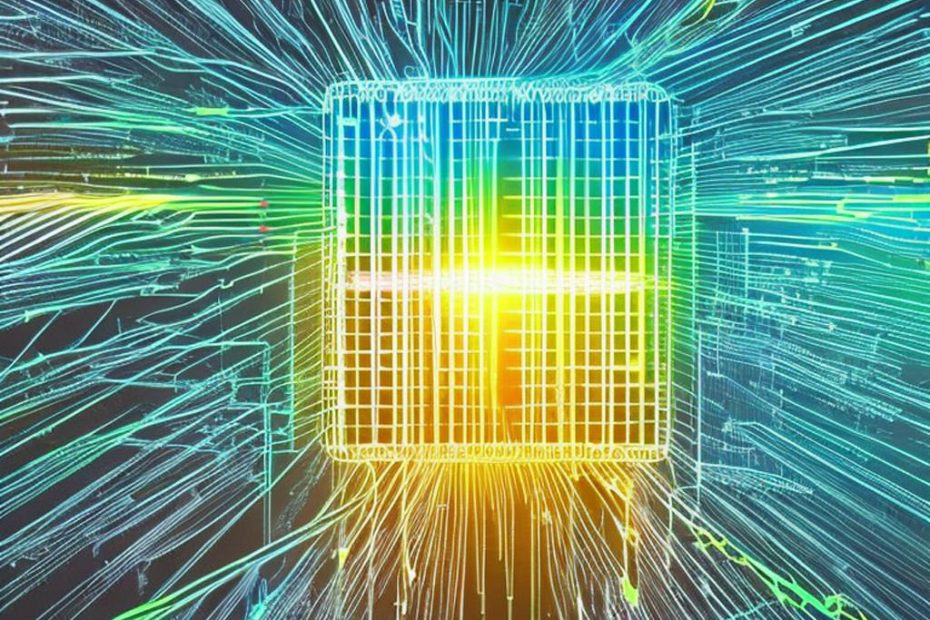# What is a quantum computer and quantum computing

• Concepts, Questions## What is a quantum computer and quantum computing

Quantum computing is a field of study that seeks to create computers that use quantum mechanical phenomena to perform operations on data. The theory behind quantum computing has been around since the early 1980s, but practical quantum computers have only recently begun to be developed. Quantum computers are fundamentally different from classical computers, which operate using classical physics, and offer the potential for dramatically faster computation in certain problem domains.

To understand what a quantum computer is and how it works, it’s necessary to first understand the basics of quantum mechanics. Quantum mechanics is the branch of physics that deals with the behavior of matter and energy on the smallest scales. At this scale, particles don’t behave like macroscopic objects; rather, they exhibit strange phenomena such as superposition and entanglement.

Superposition is the property of a quantum particle to exist in multiple states at once. For example, an electron can exist in two different energy states simultaneously. Entanglement, on the other hand, is a property of two or more particles that have interacted in such a way that their states become correlated. This means that the state of one particle is dependent on the state of the other particle, even if they are separated by large distances.

Quantum computers are based on these two phenomena. Instead of using classical bits that can only be in one of two states (0 or 1), quantum computers use quantum bits, or qubits, which can be in a superposition of states. This means that a qubit can be in both states at the same time. For example, a qubit could represent a particle that is spinning up and spinning down simultaneously.

To perform computations, quantum computers use quantum gates, which are analogous to the classical logic gates used in classical computers. Quantum gates operate on qubits, manipulating their states in a controlled way. This manipulation can be used to perform operations such as adding, subtracting, and multiplying numbers. Because of the properties of quantum mechanics, quantum gates can operate on many qubits at once, which allows quantum computers to perform certain computations much faster than classical computers.

One of the most famous algorithms for quantum computers is Shor’s algorithm for factoring large numbers. This algorithm is exponentially faster than the best known classical algorithm for factoring, which has important implications for cryptography. Other applications of quantum computing include simulating quantum systems, optimizing complex systems, and machine learning.

However, there are several challenges to building practical quantum computers. One major challenge is the issue of decoherence, which is the loss of quantum coherence due to interaction with the environment. Decoherence destroys the delicate superposition and entanglement that are necessary for quantum computation. Another challenge is the difficulty of building and controlling large numbers of qubits, which is necessary for scaling up quantum computers.

`Quantum computing is a rapidly developing field that seeks to harness the properties of quantum mechanics to create powerful computers. While there are many challenges to building practical quantum computers, the potential benefits are enormous. Quantum computers could revolutionize fields such as cryptography, machine learning, and optimization, and could lead to breakthroughs in many areas of science and technology.`

## Key points of quantum computing